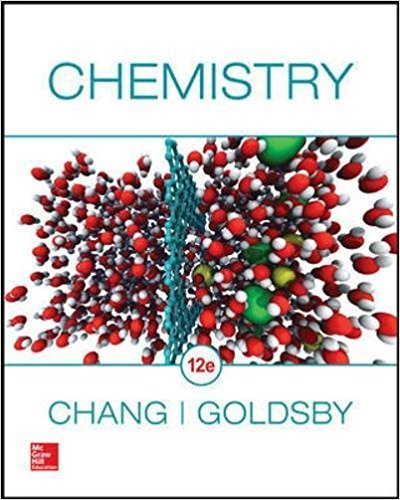×
Log in to StudySoup
Get Full Access to Chemistry - 12 Edition - Chapter 25 - Problem 25.3
Join StudySoup for FREE
Get Full Access to Chemistry - 12 Edition - Chapter 25 - Problem 25.3

Already have an account? Login here
×
Reset your password

# Answer: Calculate the molar mass of a particularISBN: 9780078021510 98

## Solution for problem 25.3 Chapter 25

Chemistry | 12th Edition

• Textbook Solutions
• 2901 Step-by-step solutions solved by professors and subject experts
• Get 24/7 help from StudySoup virtual teaching assistantsChemistry | 12th Edition

4 5 1 250 Reviews
10
4
Problem 25.3

Calculate the molar mass of a particular polyethylene sample, ( CH2CH2)n, where n 5 4600.

Step-by-Step Solution:
Step 1 of 3

Chapter 18: Aromaticity Compounds Nomenclature: - Rarely use IUPAC names - Common Examples Toluene Phenol Aniline Benzoic Acid (Methyl Benzene) (Hydroxy Benzene) Sodium Benzoate Styrene Vanillin (Vanilla) Asprin Naming: - Name using phenyl group as parent name when the side chain is not branched. If it is branched, then use the side chain as the parent. - - If benzene has CH i3 is toluene. If it has OH it is phenol. But what if it has both groups - Naming priority: CH < NH < OH < < < 3 2 R

Step 2 of 3

Step 3 of 3

##### ISBN: 9780078021510

Since the solution to 25.3 from 25 chapter was answered, more than 742 students have viewed the full step-by-step answer. The full step-by-step solution to problem: 25.3 from chapter: 25 was answered by , our top Chemistry solution expert on 09/09/17, 04:35AM. The answer to “Calculate the molar mass of a particular polyethylene sample, ( CH2CH2)n, where n 5 4600.” is broken down into a number of easy to follow steps, and 15 words. This full solution covers the following key subjects: . This expansive textbook survival guide covers 25 chapters, and 3241 solutions. This textbook survival guide was created for the textbook: Chemistry, edition: 12. Chemistry was written by and is associated to the ISBN: 9780078021510.

Unlock Textbook Solution

Enter your email below to unlock your verified solution to:

Answer: Calculate the molar mass of a particular